# PSEB 10th Class Maths Solutions Chapter 4 Quadratic Equations Ex 4.3

Punjab State Board PSEB 10th Class Maths Book Solutions Chapter 4 Quadratic Equations Ex 4.3 Textbook Exercise Questions and Answers.

## PSEB Solutions for Class 10 Maths Chapter 4 Quadratic Equations Ex 4.3

Question 1.
Find the roots of the following quadratic equations if they exist, by the method of completing the square:
(i) 2x2 + 7x + 3
(ii) 2x2 + x – 4 = 0
(ili) 4x2 + 4√3x + 3 = 0
(iv) 2x2 + x + 4 = 0
Solution:
2x2 – 7x + 3 = 0
Or 2x2 – 7x = -3
Or x2 – $$\frac{7}{2}$$x = –$$\frac{3}{2}$$
Or x2 – $$\frac{7}{2}$$x + ($$\frac{-7}{4}$$)2 = $$\frac{-3}{2}+\left(\frac{-7}{4}\right)^{2}$$

Or $$\left(x-\frac{7}{4}\right)^{2}=\frac{-3}{2}+\frac{49}{16}$$

Or $$\left(x-\frac{7}{4}\right)^{2}=\frac{-24+49}{16}$$

Or $$\left(x-\frac{7}{4}\right)^{2}=\frac{25}{16}$$

Or x – $$\frac{7}{4}$$ = $$\pm \sqrt{\frac{25}{16}}=\pm \frac{5}{4}$$

Case I:
When x – $$\frac{7}{4}$$ = $$\frac{5}{4}$$
Or x = $$\frac{5}{4}+\frac{7}{4}=\frac{5+7}{4}$$
Or x = $$\frac{12}{4}$$ = 3

Case II:
When x – $$\frac{7}{4}$$ = $$\frac{-5}{4}$$
Or x = $$\frac{-5}{4}+\frac{7}{4}=\frac{-5+7}{4}$$
Or x = $$\frac{2}{4}=\frac{1}{2}$$
Hence, roots of given quadratic equation is 3, $$\frac{1}{2}$$.2x2 + x – 4 = 0
Or 2x2 + x = 4
Or x2 + $$\frac{1}{2}$$x = $$\frac{4}{2}$$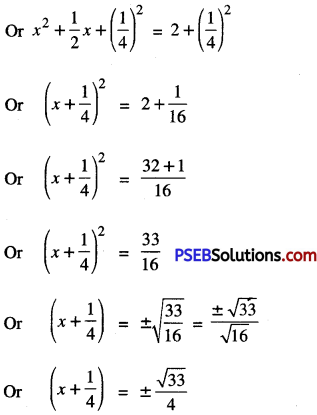Case I:
When x + $$\frac{1}{4}$$ = $$\frac{-\sqrt{33}}{4}$$
Or x = $$-\frac{\sqrt{33}}{4}-\frac{1}{4}$$
Or x = $$\frac{-\sqrt{33}-1}{4}$$
Hence, roots of given quadratic equation are $$\frac{-1+\sqrt{33}}{4}$$ and $$\frac{-1-\sqrt{33}}{4}$$.4x2 + 4√3x + 3 = 0
Or 4x2 + 4√3x = -3
Or x2 + $$\frac{4 \sqrt{3}}{4}$$x = $$\frac{-3}{4}$$
Or x2 + √3x = $$\frac{-3}{4}$$
Or x2 + √3x + $$\left(\frac{\sqrt{3}}{2}\right)^{2}=\frac{-3}{4}+\left(\frac{\sqrt{3}}{2}\right)^{2}$$
Or $$\left(x+\frac{\sqrt{3}}{2}\right)^{2}=\frac{-3}{4}+\frac{3}{4}$$
or (x + $$\frac{\sqrt{3}}{2}$$)2 = 0
(x + $$\frac{\sqrt{3}}{2}$$) (x + $$\frac{\sqrt{3}}{2}$$) = 0
Either x + $$\frac{\sqrt{3}}{2}$$ = 0
x = –$$\frac{\sqrt{3}}{2}$$
Or x + $$\frac{\sqrt{3}}{2}$$ = 0
Or x = –$$\frac{\sqrt{3}}{2}$$
Hence, roots of given quadratic equation are –$$\frac{\sqrt{3}}{2}$$ and –$$\frac{\sqrt{3}}{2}$$.

2x2 + x + 4 = 0
2x2 + x = -4
x2 + $$\frac{1}{2}$$x = $$-\frac{4}{2}$$
Or x2 + $$\frac{1}{2}$$x + ($$\frac{1}{4}$$)2 = -2 + ($$\frac{1}{4}$$)2

Or $$\left(x+\frac{1}{4}\right)^{2}=-2+\frac{1}{16}$$
Or $$\left(x+\frac{1}{4}\right)^{2}=\frac{-32+1}{16}$$
Or $$\left(x+\frac{1}{4}\right)^{2}=\frac{-31}{16}<0$$

∴ square of any number cannot be negative. So, (x + $$\frac{1}{4}$$)2 cannot be negative for any real x.
∴ There is no real x whith satisfied the given quadratic equation.
Hence, given quadratic equation has no real roots.Question 2.
Find the roots of the quadratic equations given in Q. 1 by applying the quadratic formula. Which of the above two
methods do you prefer, and why?
Solution:
2x2 – 7x + 3 = 0
Compare it with ax2 + bx + c = 0
a = 2, b = -7, c = 3
Now, b2 – 4ac = (-7)2 4 x 2 x 3
= 49 – 24
= 25 > 0
∴ x = $$\frac{-b \pm \sqrt{b^{2}-4 a c}}{2 a}$$
= $$\frac{-(-7) \pm \sqrt{25}}{2 \times 2}=\frac{7 \pm 5}{4}$$
= $$\frac{7+5}{4} \text { and } \frac{7-5}{4}$$
= $$\frac{12}{4} \text { and } \frac{2}{4}$$
= 3 and $$\frac{1}{2}$$
Hence, 3 and $$\frac{1}{2}$$ are the roots of given quadratic equation.

2x2 + x – 4 = 0
Compare it with ax2 + bx + c = 0
∴ a = 2, b = 1, c = -4
Now,
b2 – 4ac = (1)2 – 4 × 2 × 4
= 1 + 32 = 33 > 0
x = $$\frac{-b \pm \sqrt{b^{2}-4 a c}}{2 a}$$
= $$\frac{-1 \pm \sqrt{33}}{2 \times 2}=\frac{-1 \pm \sqrt{33}}{4}$$
= $$\frac{-1+\sqrt{33}}{4}$$ and $$\frac{-1-\sqrt{33}}{4}$$
Hence, $$\frac{-1+\sqrt{33}}{4}$$ and $$\frac{-1-\sqrt{33}}{4}$$ are the roots of given quadratic equation.4x2 + 4√3x + 3 = 0
Compare it with ax2 + bx + c = 0
a = 4, b = 4√3, c = 3
b2 – 4ac = (4√3)2 – 4 × 4 × (3)
= 48 – 48 = 0
∴ x = $$\frac{-b \pm \sqrt{b^{2}-4 a c}}{2 a}$$

= $$\frac{-4 \sqrt{3} \pm \sqrt{0}}{2 \times 4}$$

= $$-\frac{4 \sqrt{3}}{8}$$, $$-\frac{4 \sqrt{3}}{8}$$

= –$$\frac{\sqrt{3}}{2}$$, –$$\frac{\sqrt{3}}{2}$$

Hence, –$$\frac{\sqrt{3}}{2}$$, –$$\frac{\sqrt{3}}{2}$$ are the roots of given quadratic equation.

(iv) Given quadratic equation is 2x2 + x + 4 = 0
Compare it with ax2 + bx + c = 0
∴ a = 2, b = 1, c = 4
Now, b2 – 4ac = (1)2 – 4 × 2 × 4
= 1 – 32 = -31 < 0
But
x = $$\frac{-b \pm \sqrt{b^{2}-4 a c}}{2 a}$$
Since the square of a real number cannot be negative, therefore x will not have any real value.
Hence, there are no real roots for the given quadratic equation.

From above two questions, we used two methods to find the roots of the quadratic equations. It is very clear from above discussion that quadratic formula method is very convenient as compared to method of completing the square.Question 3.
Find the roots of the following equations:

(i) x – $$\frac{1}{x}$$ = 3, x ≠ 0
(ii) $$\frac{1}{x+4}-\frac{1}{x-7}=\frac{11}{30}$$, x ≠ -4, 7
Solution:
(i) Given Equation is
x – $$\frac{1}{x}$$ = 3
Or $$\frac{x^{2}-1}{x}$$ = 3
Or x2 – 1 = 3x
Or x2 – 3x – 1 = 0
Compare it with ax2 + bx + c = 0
∴ a = 1, b = -3, c = -1
Now, b2 – 4ac = (-3)2 – 4 . 1 . (-1)
= 9 + 4 = 13 > 0
∴ x = $$\frac{-b \pm \sqrt{b^{2}-4 a c}}{2 a}$$
= $$\frac{-(-3) \pm \sqrt{13}}{2 \times 1}$$
= $$\frac{3 \pm \sqrt{13}}{2 \times 1}$$
= $$\frac{3+\sqrt{13}}{2}$$ and $$\frac{3-\sqrt{13}}{2}$$
Hence, $$\frac{3+\sqrt{13}}{2}$$ and $$\frac{3-\sqrt{13}}{2}$$ are the roots of given quadratic equation.(ii) Given equation is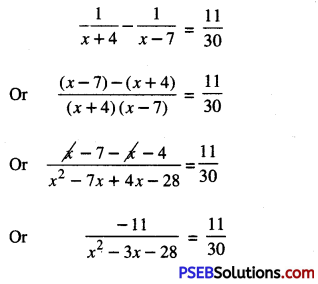-11 × 30 = 11 (x2 – 3x – 28)
Or -30 = x2 – 3x – 28
Or x2 – 3x – 28 + 30 = 0
Or x2 – 3x + 2 = 0
Compare it with ax2 + bx + c = 0
a = 1, b = – 3, c = 2
Now, b2 – 4ac = (-3)2 – 4 × 1 ×2
= 9 – 8 = 1 > 0
x = $$\frac{-b \pm \sqrt{b^{2}-4 a c}}{2 a}$$
= $$\frac{-(-3) \pm \sqrt{1}}{2 \times 1}=\frac{3 \pm 1}{2}$$
= $$\frac{3+1}{2}$$ and $$\frac{3+1}{2}$$
= $$\frac{4}{2}$$ and$$\frac{2}{2}$$ and 1
Hence, 2 and 1 are the roots of given quadratic equation.Question 4.
The sum of the reciprocals of Rehman’s age (in years) 3 years ago and 5 years from now is $$\frac{1}{3}$$. F1nd his present age.
Solution:
Let Rehman’s present age = x years
3 years ago Rehman’s age (x – 3) years
5 years from now Rehman’s age =(x + 5) years
According to question,
$$\frac{1}{x-3}+\frac{1}{x+5}=\frac{1}{3}$$

Or $$\frac{x+5+x-3}{(x-3)(x+5)}=\frac{1}{3}$$

Or $$\frac{2 x+2}{x^{2}+5 x-3 x-15}=\frac{1}{3}$$

Or $$\frac{2 x+2}{x^{2}+2 x-15}=\frac{1}{3}$$
Or 6x + 6 = x2 + 2 -15
Or x2 + 2x – 15 – 6x – 6 = 0
Or x2 – 4x – 21 = 0, which is quadratic in x.
So compare it with ax2 + bx + c =0
a = 1, b = -4, c = -21
Now, b2 – 4ac = (- 4)2 4 × 1 × (-21)
= 16 + 84 = 100 > 0
∴ x = $$\frac{-b \pm \sqrt{b^{2}-4 a c}}{2 a}$$
x = $$\frac{-(-4) \pm \sqrt{100}}{2 \times 1}$$
= $$\frac{4 \pm 10}{2}$$
= $$\frac{4+10}{2}$$ and $$\frac{4-10}{2}$$
$$\frac{14}{2}$$ and $$\frac{-6}{2}$$
= 7 and -3
∵ age cannot be negative,
so, we reject x = – 3
∴ x = 7
Hence, Rehman’s present age = 7 years.Question 5.
In a class test, the sum of Shefall’s marks in Mathematics and English is 30. Had she got 2 marks more in Mathematics and 3 marks less ¡n English, the product of their marks would have been 210. Find her marks In the two subjects.
Solution:
Let Shefali get marks in Mathematics = x
Shefali’s marks in English = 30 – x
According to 1st condition,
Shefali’s marks in Mathematics = x + 2
and Shefali’s marks in English = 30 – x – 3 = 27 – x
∴ Their product = (x + 2) (27 – x)
= 27x – x2 + 54 – 2x
= x2 + 25x + 54
According to 2nd condition,
-x2+ 25x+ 54 = 210
Or -x2 + 25x + 54 – 210 = 0
Or -x2 + 25x – 156 = 0
Or x2 – 25x+ 156 = o
Compare it with ax2 + bx + c = O
a = 1, b = -25, c = 156
Now, b2 – 4ac = (-25)2 – 4 × 1 × 156
= 625 – 624 = 1 > 0
x = $$\frac{-b \pm \sqrt{b^{2}-4 a c}}{2 a}$$
= $$\frac{-(-25) \pm \sqrt{1}}{2 \times 1}$$
= $$\frac{25 \pm 1}{2}$$
= $$\frac{25+1}{2}$$ and $$\frac{25-1}{2}$$
= $$\frac{26}{2}$$ and $$\frac{24}{2}$$
= 13 and 12.

Case I:
When x = 13
then Shefaiis marks in Maths = 13
Shefali’s marks in English = 30 – 13 = 17.

Case II:
When x = 12
then Shefalis marks in Maths = 12
Shefali’s marks in English = 30 – 12 =18.
Hence, Shefalis marks in two subjects are 13 and 17 Or 12 and 18.Question 6.
The diagonal of a rectangular field is 60 metres more than the shorter side. if the longer side is 30 metres more than the shorter side, find the sides of the field.
Solution:
Let shorter side of rectangular field = AD = x m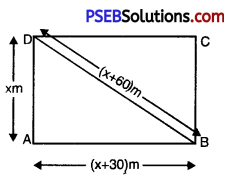Longer side of rectangular field = AB = (x + 30) m
and diagonal of rectangular field = DB = (x + 60) m
In rectangle. the angle between the length and breadth is right angle.
∴ ∠DAB = 90°
Now, in right angled triangle DAB, using Pythagoras Theorem,
(x + 60)2 = (x)2 + (x + 30)2
Or x2 + 3600 + 120x = x2 + x2 + 900 + 60x
Or x2 + 3600 + 120x – 2x2 – 900 – 60x = 0
Or -x2 + 60x + 2700 = 0
Or x2 – 60x – 2700 = 0
Compare it with ax2 + bx + e = O
∴ a = 1, b = -60, c = -2700
and b2 – 4ac = (-60)2 – 4. 1 . (-2700)
= 3600 + 10800 = 14400 > 0
∴ x = $$\frac{-b \pm \sqrt{b^{2}-4 a c}}{2 a}$$
= $$\frac{-(-60) \pm \sqrt{14400}}{2 \times 1}$$

= $$\frac{60 \pm 120}{2}$$

= $$\frac{60+120}{2}$$ and $$\frac{60-120}{2}$$
= $$\frac{180}{2}$$ and $$\frac{-60}{2}$$
= 90 and – 30
∴ length of any side cannot be negative
So, we reject x = -30
∴ x = 90
Hence, shorter side of rectangular field = 90 m
Longer side of rectangular field = (90 + 30) m = 120 m.Question 7.
The difference of squares of two numbers is 180. The square of the smaller number is 8 times the larger number. Find
the two numbers.
Solution:
Let larger number = x .
Smaller number = y
According to 1st condition,
x2 – y2 = 180 ……………(1)
According to 2nd condition,
y2 = 8x
From (1) and (2), we get
x2 – 8x = 180
Or x2 – 8x – 180 = 0
Compare it with ax2 + bx + c = 0
∴ a = -1, b = -8, c = -180
and b2 – 4ac = (-8)2 – 4 × 1 × (-180)
= 64 + 720 = 784 > 0
∴ x = $$\frac{-b \pm \sqrt{b^{2}-4 a c}}{2 a}$$
= $$\frac{-(-8) \pm \sqrt{784}}{2 \times 1}$$
= $$\frac{8 \pm 28}{2}$$
= $$\frac{8+28}{2}$$ and $$\frac{8-28}{2}$$
= $$\frac{36}{2}$$ and $$\frac{-20}{2}$$
= 18 and -10
When x = – 10 then from (2),
y2 = 8 (- 10) = – 80, which is impossible.
So, we reject x = – 10
When x = 18 then from (2).
y2 = 8(18) = 144
Or y = ±√144
Or y = ± 12
Hence, required numbers are 18 and 12 Or 18 and -12.Question 8.
A train travels 360 km ¡t a uniform speed. If the speed had been 5 km/h more, it would have taken 1 hour less for the same journey. Find the speed of the train.
Solution:
Let constant speed of the train = x km/hour
Distance covered by the train = 360 km
Time taken by the train = $$=\frac{\text { distance }}{\text { speed }}$$
(∵ speed = $$\frac{\text { Distance }}{\text { Time }}$$)
= $$\frac{360}{x}$$
Increased speed of the train = (x + 5) km/hour
∴ Time taken by the train with increased speed = $$\frac{360}{x+5}$$ hour
According to question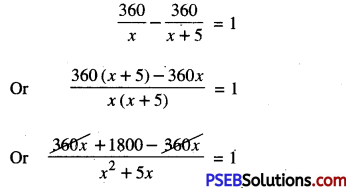Or 1800 = x2 + 5x
Or x2 + 5x – 1800 = 0
Compare it with, ax2 + bx + c = 0
a = 1, b = 5, c = – 1800
and b2 – 4ac = (5)2 4 × 1 × (- 1800)
= 25 + 7200 = 7225 > 0

x = $$\frac{-b \pm \sqrt{b^{2}-4 a c}}{2 a}$$
= $$\frac{-5 \pm \sqrt{7225}}{2 \times 1}$$
= $$\frac{-5 \pm 85}{2}$$
= $$\frac{-5+85}{2}$$ and $$\frac{-5-85}{2}$$
= $$\frac{80}{2}$$ and $$\frac{-90}{2}$$
= 40 and – 45
∵ speed of any train cannot be negative.
So, we reject x = – 45
x = 40
Hence, speed of train = 40 km/hour.Question 9.
Two water taps together can fill a tank in 9$$\frac{3}{8}$$ hours. The tap of larger diameter takes 10 hours less than the smaller one to fill the tank separately. Find the time in which each tap can separately fill the tank.
Solution:
Let time taken by larger tap to fill the tank = x hours.
Time taken by smaller tap to fill the tank = (x + 10) hours
In case of one hour:
Larger tap can fill the tank = $$\frac{1}{x}$$
Smaller tap can fill the tank = $$\frac{1}{x+10}$$
∴ Larger and smaller tap fill the tank = $$\frac{1}{x}$$ + $$\frac{1}{x+10}$$ ………….(1)
But, two taps together can fill the tank = 9$$\frac{3}{8}$$hour = $$\frac{75}{8}$$ hour
Now, two taps together can fill the tank in one hour = $$\frac{8}{75}$$ ……………..(2)
From (1) and (2), we get
$$\frac{1}{x}+\frac{1}{x+10}=\frac{8}{75}$$

Or $$\frac{x+10+x}{x(x+10)}=\frac{8}{75}$$

Or $$\frac{2 x+10}{x^{2}+10 x}=\frac{8}{75}$$

Or 75(2x + 10) = 8(x2 + 10x)
Or 150x + 750 = 8x2 + 80x
Or 8x2 + 80x – 150x – 750 = 0
Or 8x2 – 70x – 750 = 0
Or 4x2 – 35x – 375 = 0
Compare it with ax2 + bx + c = 0
∴ a = 4, b = -35, c = -375
and b2 – 4ac = (35)2 -4 × 4 × (-375)
= 1225 + 6000 = 7225 > 0
∴ x = $$\frac{-b \pm \sqrt{b^{2}-4 a c}}{2 a}$$

= $$\frac{-(-35) \pm \sqrt{7225}}{2 \times 4}$$

= $$\frac{35 \pm 85}{8}$$

= $$\frac{35+85}{8}$$ and $$\frac{35-85}{8}$$

= $$\frac{120}{8}$$ and $$\frac{-50}{8}$$

= 15 and $$\frac{-25}{4}$$

∵ time cannot be negative.
So,we reject x = $$\frac{-25}{4}$$
∴ x = 15
Hence, larger water tap fills the tank = 15 hours
and smaller water tap fills the tank = (15 + 10) hours = 25 hours.Question 10.
An express train takes 1 hour less than a passenger train to travel 132 km between Mysore and Bangalore (without taking into consideration the time they stop to intermediate stations), if the average speed of the express train is 11 km/hr more than that of the passenger train, find the average speed of the two trains.
Solution:
Let average speed of passenger train = x km/hour
Average speed of express train = (x+ 11) km/hour
Distance between Mysore and Bangalore = 132 km
Time taken by passenger train = $$\frac{132}{x}$$ hour
[∵ Speed = $$=\frac{\text { Distance }}{\text { Time }}$$ ]
Time taken by express train‚ = $$\frac{132}{x+11}$$ hour
According to question,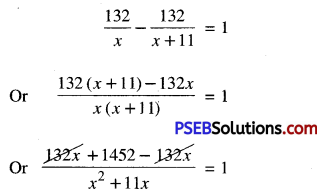Or 1452 = x2 + 11x
Or x2 + 11x – 1452 = 0
Compare it with ax2 + bx + c = 0
∴ a = 1, b = 11, c = -1452
and b2 – 4ac = (11)2 – 4 × 1 × (- 1452)
= 121 + 5808 = 5929 > 0
∴ x = $$\frac{-b \pm \sqrt{b^{2}-4 a c}}{2 a}$$
= $$\frac{-11 \pm \sqrt{5929}}{2 \times 1}$$

= $$\frac{-11 \pm 77}{2}$$

= $$\frac{-11+77}{2}$$ and $$\frac{-11-77}{2}$$

= $$\frac{66}{2}$$ and $$\frac{-88}{2}$$ = 33 and -44

∵ speed of any train cannot be negative
∴ x = 33
Hence, speed of passenger train = 33 km/hour
and speed of express train = (33 + 11) km/hour = 44 km/hour.Question 11.
Sum of the areas of two squares is 468 m2. If the difference of their perimeters
is 24 m, find the sides of the two squares.
Solution:
In case of larger square
Let length of each side of square = x m
Area of square = x2 m2
Perimeter of square = 4x m

In case of smaller square:

Let lenth of each side of square = y m
Area of square = y2 m2
Perimeter of square = 4y m
According to 1st condition,
x2 + y2 = 468 …………….(1)
According to 2nd condition,
4x – 4y = 24
Or 4(x – y) = 24
Or x – y = 6
x = 6 + y
From (1) and (2), we get
(6 + y)2 + y2 = 468
Or 36 + y2 + 12y + y2 = 468
Or 2y2 + 12y + 36 – 468 = 0
Or 2y2 + 12y – 432 = 0
Or y2 + 6y – 216 = 0
Compare it with ay2 + by + c = 0
∴ a = 1, b = 6, c = -216
and b2 – 4ac = (6)2 – 4 × 1 × (- 216) = 36 + 864 = 900 > 0
∴ y = $$\frac{-b \pm \sqrt{b^{2}-4 a c}}{2 a}$$

= $$\frac{-6 \pm \sqrt{900}}{2 \times 1}$$

= $$\frac{-6 \pm 30}{2}$$

= $$\frac{-6+30}{2}$$ and $$\frac{-6-30}{2}$$

= $$\frac{24}{2}$$ and $$\frac{-36}{2}$$ = 12 and -18

∵ length of square cannot be negative
So, we reject y = – 18
∴ y = 12
From (2), x = 6 + 12 = 18
Hence, sides of two squares are 12 m and 18 m.